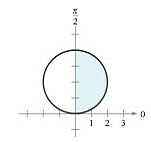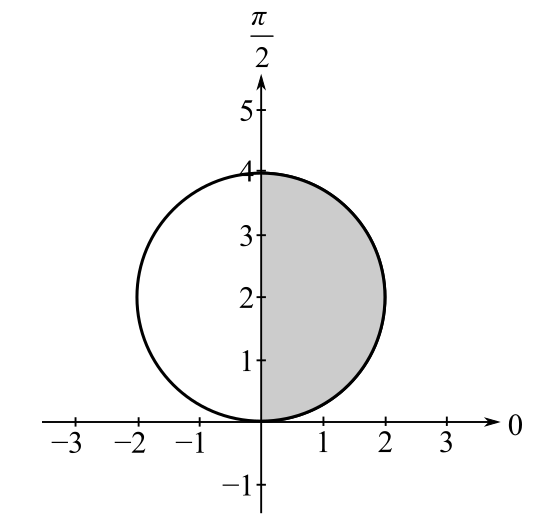Chapter 10.5, Problem 3E

Chapter
Section
Textbook Problem

Area of a Polar Region In Exercises 3-6, write an integral that represents the area of the shaded region of the figure. Do not evaluate the integral. r = 4 sin θTo determine

To calculate: The integral that represents the area of shaded region of the figure:Explanation

Given:

The polar equation is r=4sinθ and graph is shown as below:

Formula used:

The area of polar co-ordinates is A=12αβr2dθ.

Calculation:

From the above graph it is clear that shaded region lies in first quadrant over which angle θ lies in the range from 0 to π2.

The provided polar equation is r=4sinθ

Still sussing out bartleby?

Check out a sample textbook solution.

See a sample solution

The Solution to Your Study Problems

Bartleby provides explanations to thousands of textbook problems written by our experts, many with advanced degrees!

Get Started

Solve the inequality. 48. |x| 3

Single Variable Calculus: Early Transcendentals, Volume I

For the following set of scores, find the value of each expression: a. X b. (X)2 c. X2 d. (X +3)

Essentials of Statistics for The Behavioral Sciences (MindTap Course List)

In problems 45-62, perform the indicated operations and simplify. 49.

Mathematical Applications for the Management, Life, and Social Sciences

Solve each equation and check: 2x=6

Elementary Technical Mathematics

For f(x) = 6x4, f′(x) = _____. 24x 6x3 10x3 24x3

Study Guide for Stewart's Single Variable Calculus: Early Transcendentals, 8th